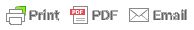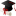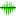80s toys - Atari. I still have

Neco RunsWaec RunsJamb RunsNabteb RunsTop FeedsForum PortalHomepage

Chat ( 1 )

Sunday December 3rd 2023

Do You Have A Question to ask?

MATHS SYMBOLS FOR OUR RUNS

Posted on April 17th, 2018 by Examsolution
This is how we send our Maths Symbols and the way you are suppose to write it on ur paper.

MATHS SYMBOLS

(1) / means division or divide
Examples:
2/2 means 2/2
2 whole no 3/4 means 2¾
M=wp (1/3)yp^2 means m=wp ¾y 2

(2) Log means logarithm Example: (8) Log5 (base 8) means Log8 5

(3) * means multiplication Example: 2*2 means 2×2

(4) ^ means Raise to power
Examples:
3^(-1) means 3 -1
5^(2) means 5 2
30cm^(-2) means 30cm -2

(5) Tita means θ
Example: Tan tita means tan θ

(6) Pie means π
Example: Pie R sqr h means πr 2 h

(7) Base means subscript
Example: 2 Base 3 means A 3

(8) Sqr root means √
Examples:
√3-√6 2√3 means Root 3-root6 2root 3
√(¾) means sqr root(3/4)

(9) bar means a dash on top a number of letter
Example: X bar means x̅

(10) —(1) means equation 1

(11) Proportional means ∝
Example: R is proportional to 3/4rut m means R
∝ ¾

(12) Alpha means α

(13) Beta means β

(14) Gamma means γ

(15) cube root means ∛

(16) Mu means μ

(17) Rho means ρ

(18) Delta means δ

(19) Sigma means σ

(20) Tau means τ

(21) Ohm means Ω

(22) Lamda means λ

(23) Omega means ω

(24) Intersection means ∩
Example: B intersection C means B∩C

(25) Union means U
Example: B union C means BUC

(26) Factorial means !

(27) complements in sets means ‘

(28) (aq),(g),(l),(s) is used in chemistry to show the state of matter in equations

(29) Equivalent to means ≡

(30) Not equal to means ≠

(31) Quotation means “ ”

(32) Less or equal to means ≤

(33) Greater or equal to ≥
Example: (3/4)>=(1/2) means ¾ ≥ ½

(34) Plus or minus (or -) means ±
Example: (3/4)<=(1/2) means ¾ ≤ ½

Share and Invite Ur Friends Now«Back©CAMPUSBASE .INC

PROJECT BY: Sir ExamsolutionRemita:
872